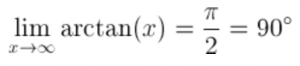# Arctan of infinity

What is the arctangent of infinity and minus infinity?

arctan(∞) = ?

The arctangent is the inverse tangent function.

The limit of arctangent of x when x is approaching infinity is equal to pi/2 radians or 90 degrees:The limit of arctangent of x when x is approaching minus infinity is equal to -pi/2 radians or -90 degrees: